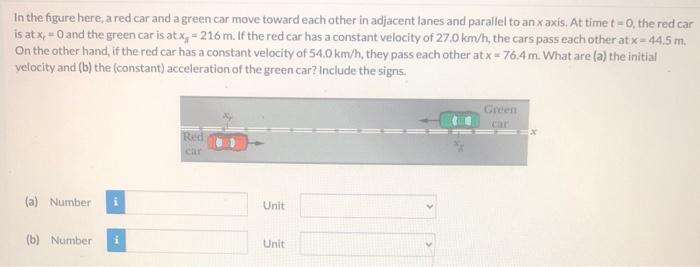# (Solved): In the figure here, a red car and a green car move toward each other in adjacent lanes and parallel ...In the figure here, a red car and a green car move toward each other in adjacent lanes and parallel to an $$x$$ axis. At time $$t=0$$, the red car is at $$x_{p}=0$$ and the green car is at $$x_{\mathrm{g}}=216 \mathrm{~m}$$. If the red car has a constant velocity of $$27.0 \mathrm{~km} / \mathrm{h}$$, the cars pass each other at $$x=44.5 \mathrm{~m}$$. On the other hand, if the red car has a constant velocity of $$54.0 \mathrm{~km} / \mathrm{h}$$, they pass each other at $$x=76.4 \mathrm{~m}$$. What are (a) the initial velocity and (b) the (constant) acceleration of the green car? Include the signs,

We have an Answer from Expert